Algebra 1 3-5 Guided Practice: Working With Sets
starstarstarstarstarstarstarstarstarstar
by Matthew Richardson
| 22 Questions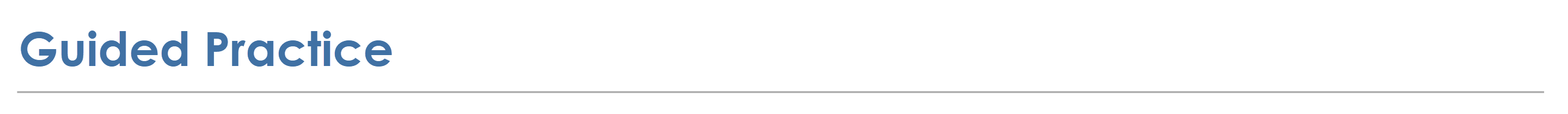1
1
10
Solve It! Most numbers on a telephone keypad correspond to a set of letters. Suppose you're friend is using the keypad to encrypt a secret message. He sends you the string of characters 4 6 6 3 in that order. What is one word he might be trying to send?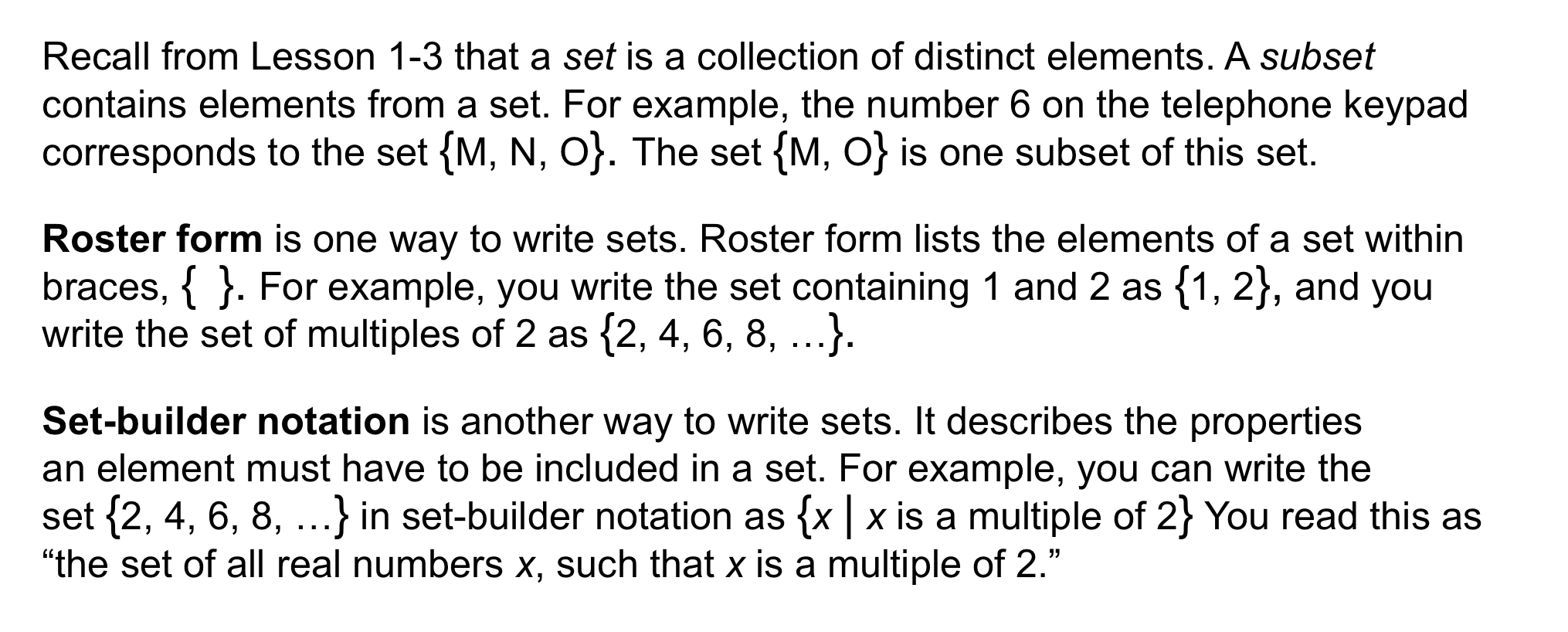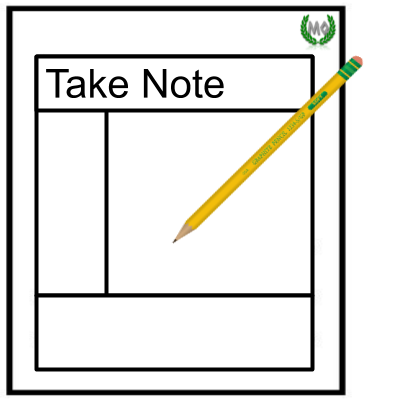2
3
4
5
2
10
Take Note: Define set as it pertains to this lesson.
3
10
Take Note: Define subset as it pertains to this lesson.
4
5
Take Note: Provide an example of a set containing 5 elements that is written in roster form.
5
5
Take Note: Provide an example of a set that is written using set-builder notation.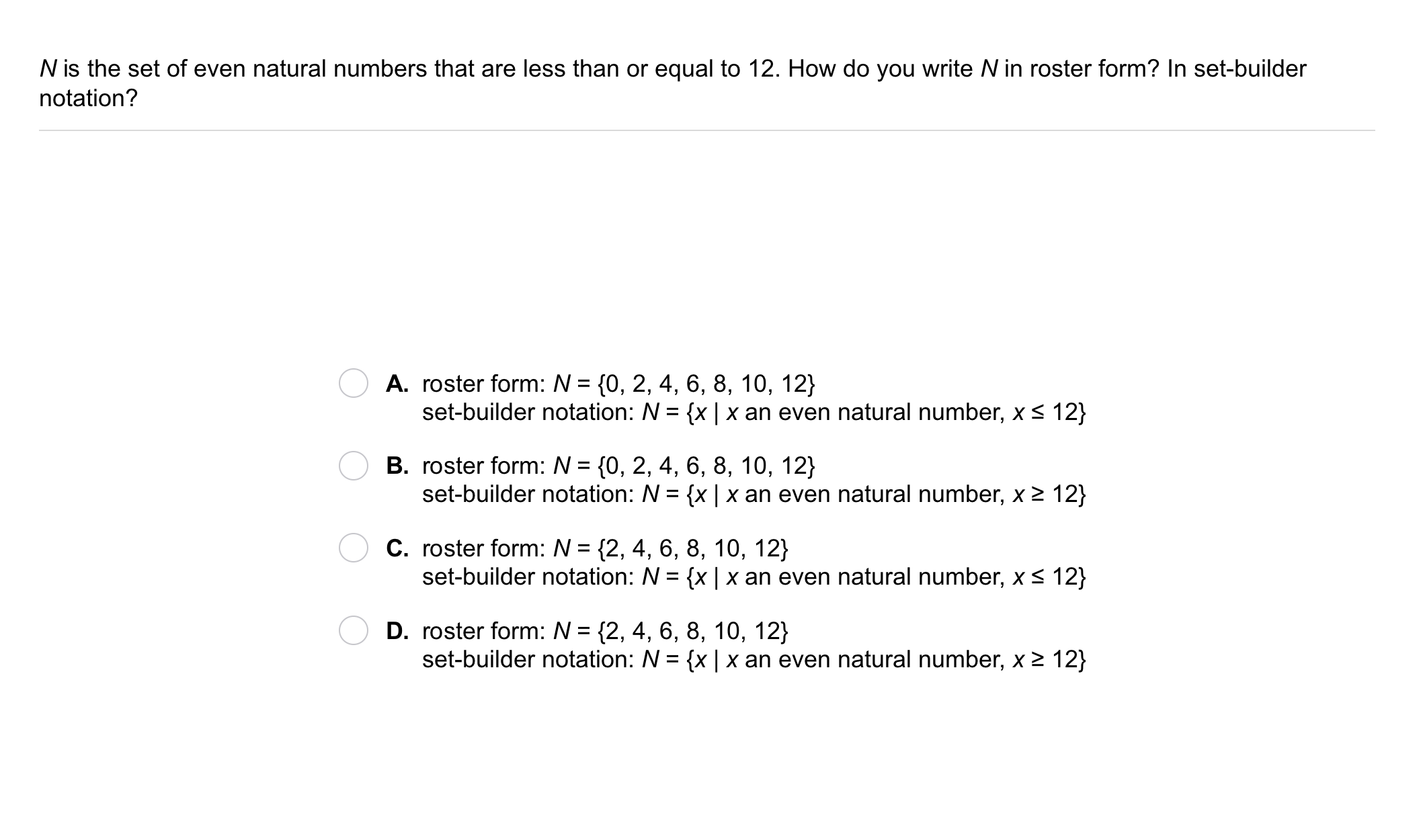6
6
10
Problem 1 Got It?
A
B
C
D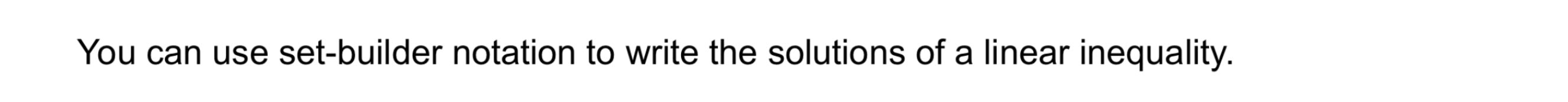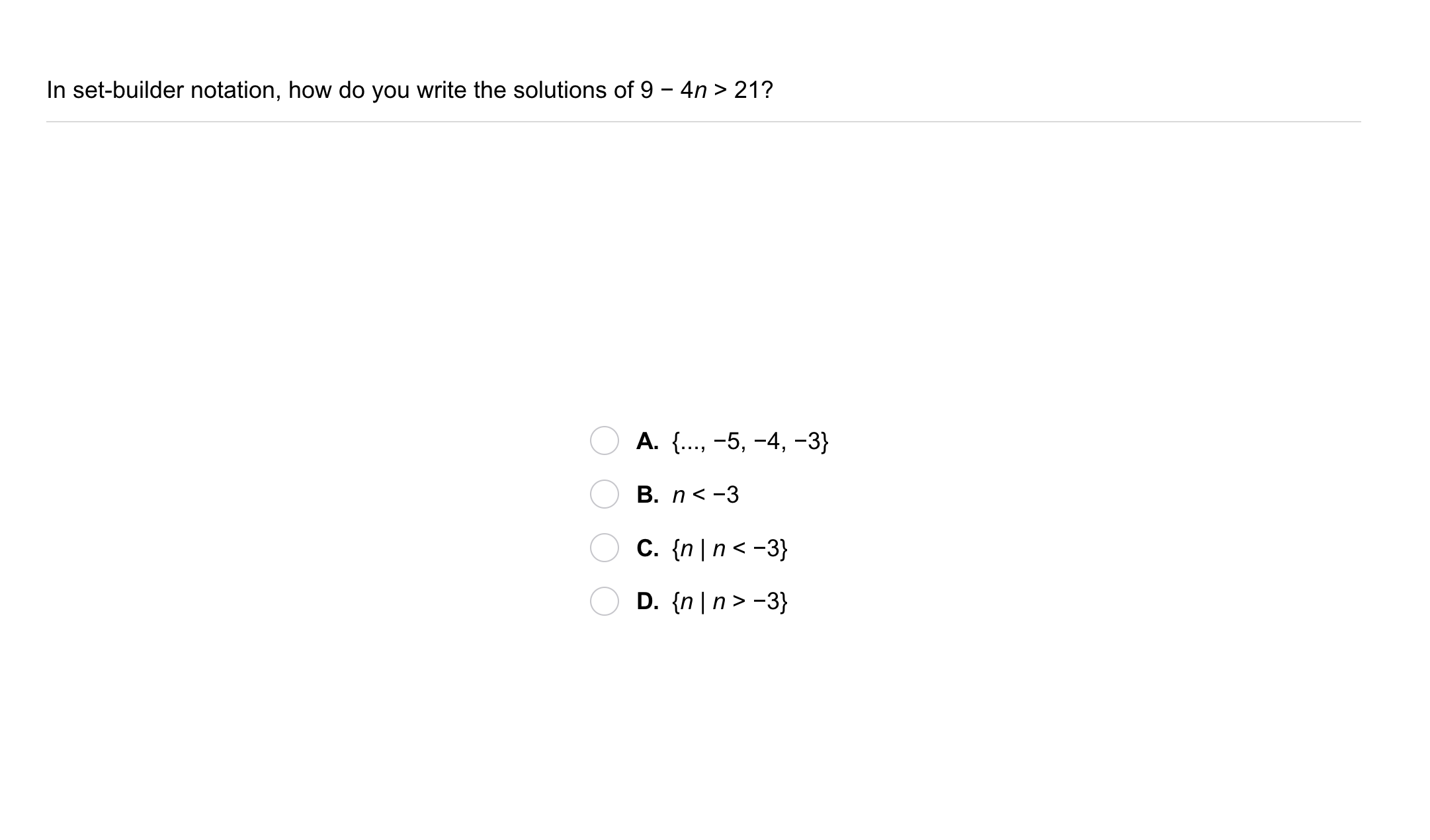7
7
10
Problem 2 Got It?
A
B
C
D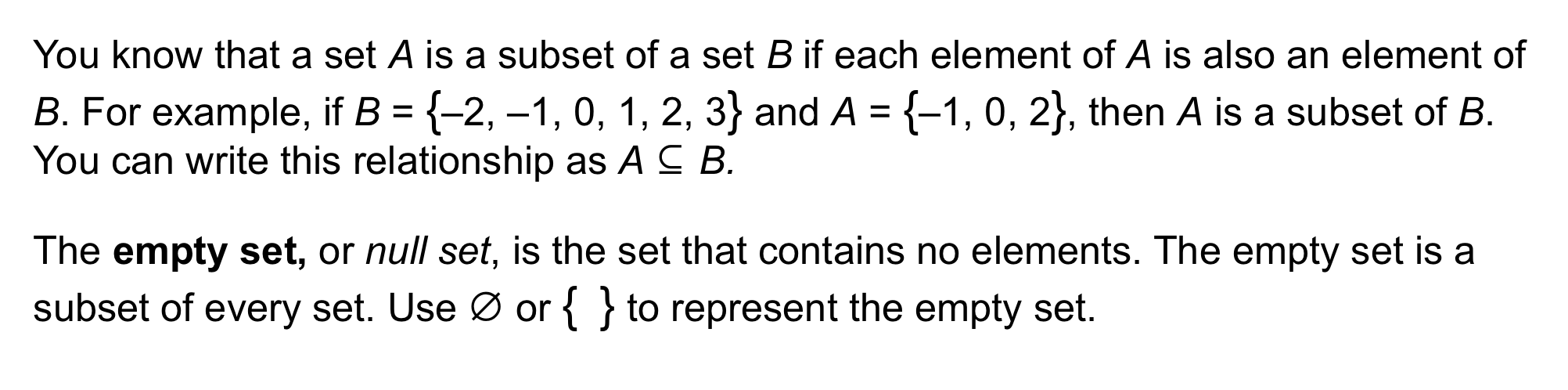8
9
10
11
12
13
8
10
Take Note: Define subset.
9
5
Take Note: Provide an example of two sets written in roster form. The second set should be a subset of the first.
10
5
Take Note: Every set is a subset of itself.
True
False
11
10
Take Note: Define the empty set.
12
5
Take Note: Write the empty set in roster form.
13
10
Take Note: Mathtype pro-tip!

In many equation editors, the code \subseteq will generate the subset symbol ⊆. You can try it out here.

Enter the subset symbol.
14
10
Problem 3 Got It? What are the subsets of the set P = {a, b}?
Select all that apply.
{a, b}
{a}
{} (aka ø or the empty set)
{b}
15
10
Problem 3 Got It? What are the subsets of the set S = {a, b, c}?
Select all that apply.
{b}
{b, c}
{c}
{a}
{a, c}
{d}
{} (aka ø or the empty set)
{a, b}
{a, b, c}
16
5
Problem 3 Got It? Reasoning: Let A = {x | x < -3} and B = {x | x ≤ 0}. Is A a subset of B?
Yes
No
17
10
Problem 3 Got It? Reasoning: Explain your reasoning on the previous item.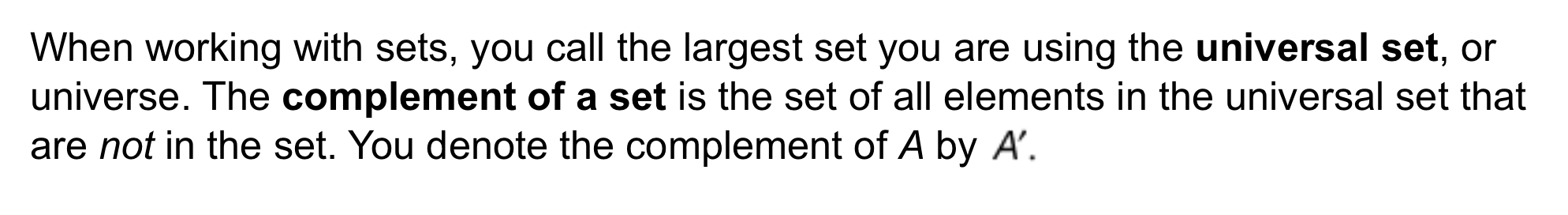18
19
20
18
10
Take Note: Define universal set (aka universe).
19
10
Take Note: Define complement of a set.
20
10
Take Note: Let the universal set U and a set A be defined as below.

U = {1,2,3,4,5}
A = {1,3,5}

Write A' in roster form.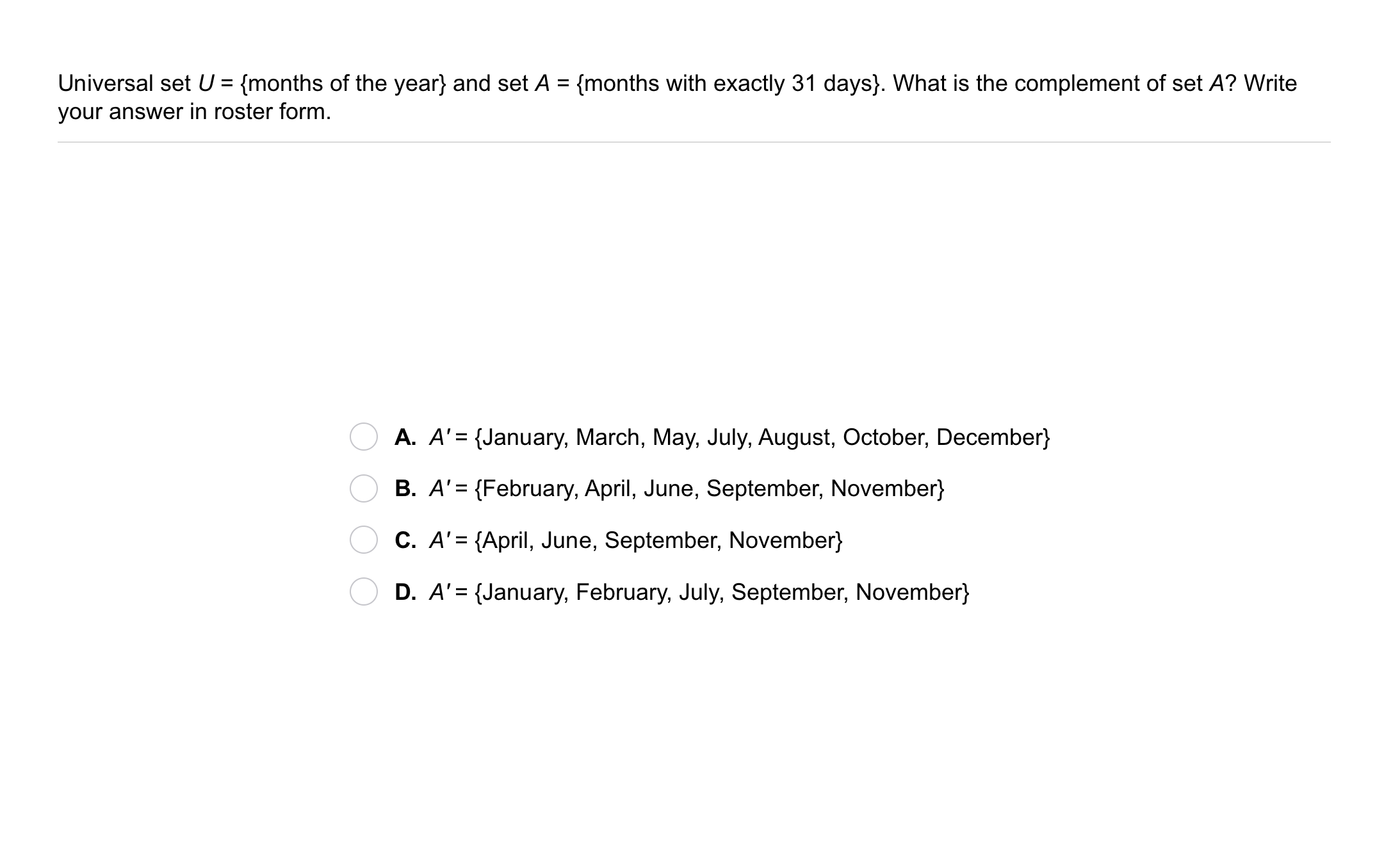21
21
10
Problem 4 Got It?
A
B
C
D22
22
10
Take Note: Summarize the mathematical content of this lesson. What topics, ideas, and vocabulary were introduced?